﻿ The Asymptotic Noise Distribution in Karhunen-Loeve Transform Eigenmodes
Journal of Health & Medical Informatics

Like us on:

All submissions of the EM system will be redirected to Online Manuscript Submission System. Authors are requested to submit articles directly to Online Manuscript Submission System of respective journal.
• Research Article
• J Health Med Inform 2013, Vol 4(2)
• DOI: 10.4172/2157-7420.1000122

# The Asymptotic Noise Distribution in Karhunen-Loeve Transform Eigenmodes

Yu Ding1,2, Hui Xue3*, Ning Jin4, Yiu-Cho Chung2, Xin Liu2, Yongqin Zhang2 and Orlando P. Simonetti1,5,6,7
1Davis Heart and Lung Research Institute, The Ohio State University, Columbus, USA
2Shenzhen Institute of Advanced Technology of Chinese Academy of Science, Shenzhen, Guangdong, China
3Siemens Corporate Research, Princeton, USA
4Siemens Medical Solutions, Inc., Columbus, USA
5Department of Internal Medicine, The Ohio State University, Columbus, USA
6Department of Biomedical Engineering, The Ohio State University, Columbus, USA
7Department of Radiology, The Ohio State University, Columbus, USA
*Corresponding Author: Hui Xue, Siemens Corporation, Corporate Research, 755 College Road East, Princeton, NJ 08540, USA, Tel: +1(609)734-6560, +1(609)712-3398, Fax: +1(609)734-6565, Email: [email protected]

Received Date: Dec 01, 2012 / Accepted Date: Feb 01, 2013 / Published Date: Feb 03, 2013

### Abstract

Karhunen-Loeve Transform (KLT) is widely used in signal processing. Yet the well-accepted result is that, the noise

is uniformly distributed in all eigenmodes is not accurate. We apply a result of the random matrix theory to understand
the asymptotic noise distribution in KLT eigenmodes. Noise variances in noise-only eigenmodes follow the Marcenko-
Pastur distribution, while noise variances in signal-dominated eigenmodes still follow the uniform distribution. Both the
mathematical expectation of noise level in each eigenmode and an analytical formula of KLT filter noise reduction effect
with a hard threshold were derived. Numerical simulations agree with our theoretical analysis. The noise variance of
an eigenmode may deviate more than 60% from the uniform distribution. These results can be modified slightly, and
generalized to non-IID (independently and identically-distributed) noise scenario. Magnetic resonance imaging experiments
show that the generalized result is applicable and accurate. These generic results can help us understand the
noise behavior in the KLT and related topics.

Keywords: Karhunen-Loeve transform; Random matrix theory; Independently and identically-distributed noise

#### Introduction

Karhunen-Loeve Transform (KLT, a.k.a. principal component analysis or singular value decomposition) is an important tool in dimension reduction, data mining, and denoising [1,2]. It transforms the original data set into a series of orthogonal eigenmodes with eigenvalues λ1 ≤ λ2 ≤ … ≤ λm. It is believed that when a data set is corrupted by additive independently and identically-distributed (IID) noise with variance σ2, the unitarity of the KLT ensures that the noise in each eigenmode is also white with variance σ2 [3,4,5]. Based on this assumption, the noise variance can be estimated from the smallest eigenvalue λ1 . Furthermore, a threshold can be applied safely to remove all noise-only eigenmodes, eg. truncate all eigenmodes with λi ≤ σ2 (assume σ2 is known) in order to suppress noise using a KLT filter [5,7].

However, the noise variance in KLT eigenmodes may not be uniformly distributed. When noise-only eigenmodes appear, a theoretical result of the random matrix theory (RMT)  predicts that the corresponding eigenvalues follow the Marcenko-Pastur (MP) distribution [9,10], instead of the uniform distribution. Therefore, the “common sense” of the noise distribution in KLT eigenmodes mentioned above may not be accurate enough. In this communication, we apply this RMT result to study the noise variance distribution in KLT eigenmodes. We show that the variances in noise-only eigenmodes follow the MP distribution, while noise variances in signal-dominated eigenmodes still follow the uniform distribution. In addition, the smallest eigenvalue λ1 could be much lower than σ2, and the eigenvalues of some noise-only eigenmodes may be much higher than σ2. Based on this result, first, we derive the mathematical expectation of the noise level in each eigenmode; second, we derive an analytical relation between the threshold or filter cutoff and the noise level in the KLT filtered data set. However, IID noise is rarely the case in practice. Noise usually has some degree of correlation and amplitude variation. Therefore, MP distribution is not applicable. According to our previous study, the MP distribution can be modified slightly by re-define a parameter, and become applicable to some important non-IID noise scenario . Numerical simulations are used to validate our results, and real-time MR cardiac cine images are used to show that our results can be utilized in experimental data analysis. Therefore, our research helps clarify theoretically the noise reduction effect of KLT-based filter used in medical imaging society [5,7], and provides an explanation why the random matrix theory based noise level estimation  is better than smallest eigenvalue based method .

#### Theory

Additive independently and identically-distributed noise

We assume that a data set can be represented by an m by n matrix A, without loss of generality, m<n. A can be regarded as a sum of a low rank signal matrix S and a random matrix N with IID noise (variance=σ2) in each entry:

A=S + N (1)

The rank of S is r, and r<m. In other words, there is intrinsic redundancy in S and A. The empirical covariance matrix R of A, defined as AAT/n (ATrepresents the transpose of A) is full rank. When the smallest non-zero eigenvalue of the covariance matrix of S satisfies λmin≥ σ2√(d/n), the Probability Distribution Function (PDF) of the smallest d (where d=m-r) eigenvalues of R follows the Marcenko- Pastur (MP) distribution from the RMT [9,10]: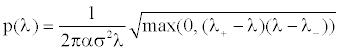(2)

Where α=d/n,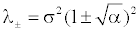Equation 2 is an asymptotic result when n,d >>1 (from our experience n,d>10 is sufficient, in agreement with previously published observations . The first d eigenmodes are noise-only (eigenmodes are sorted in ascending order by eigenvalues); eigenvalues λi (i=1, …, d) are the noise variances of the corresponding eigenmodes. Therefore, the noise variance PDF of the first d eigenmodes follows equation 2. Please refer to equation A.2 in Appendix for the mathematical expectation of the noise level in each eigenmode. The smallest eigenvalue λ1is lower than σ2. When α~1, λ1 is a poor estimation of σ21<<σ2).

The last r eigenmodes are signal-dominated. Because signal is uncorrelated with noise and due to the unitarity of KLT, noise variances are identical (=σ2) in the last r eigenmodes. Therefore, noise variances in first d eigenmodes follow the MP distribution, while noise variances in last r eigenmodes follow the uniform distribution.

The KLT filter can be applied by using a threshold to truncate some eigenmodes, and then reconstructing the filtered matrix Â using the remaining eigenmodes. Therefore, Â is a low-rank approximation of A, and is optimal in the 2-norm sense [5,13]. The noise variance distribution in eigenmodes can describe the noise variance in Â when the eigenvalue threshold=λc. Suppose k eigenmodes with eigenvalues ≤ λc are truncated. The mean noise variance f(k) of KLT filtered Â is: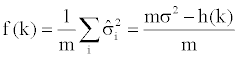(3)

where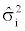is the mean noise variance of the ith row of Â; h(k) is the total noise variance in k truncated eigenmodes. In order to deduce Equation 3, we take advantages of two properties: first, the KLT is unitary; second, the noise variance is additive. When λc ≥ λ+,

h(k)=kσ2 (4a)

When λc+,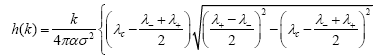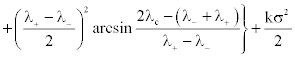(4b)

Please refer to the Appendix for more details.

When α→0, p(λ)→δ(λ−σ2), (4b) is identical to (4a), and (3) becomes: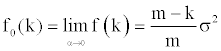(5)

Additive Non-IID Noise

The MP-law is only applicable to additive IID noise in rank deficit data matrix A. If matrix N is non-IID additive noise, then the noise covariance of matrix N become a fourth order tensor Cij,kl, where (i, j) and (k, l) are indices of two data points in matrix A. If the noise is IID, tensor Cij,kl degenerates into the multiplication of two identity matrices: σ2δijδkl, where σ2 is the noise variance, and δij is the Kronecker delta. Our recent study found that the MP-law is still applicable when the noise covariance tensor can be written as: Dijδkl, where D is a generic 2-D covariance matrix. In other words, the noise is IID only in one dimension. In practice, this is a very common scenario when the noise has spatial correlations but no temporal correlations, such as Magnetic Resonance (MR) dynamic imaging. It has been shown that a modified MP-law is still applicable in dynamic MR images, i.e. equation 2 is still accurate enough with modified parameters: n→n’, α→α’=d/n’,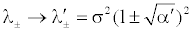. Hence, the rest of the equations are also applicable because they are derived from equation 2. Please note that the application of modified MP distribution to non-IID noise scenario is only an empirical approximation, a strict mathematical proof is warranted.

#### Methods

We tested the effectiveness of equations 2-4, using numerical simulations and real-time dynamic MR cardiac imaging in a volunteer. The human study was approved by The Ohio State University’s Human Subjects Committee and all subjects gave written informed consent to participate. The volunteer images were acquired on a 3.0T MRI system (MAGNETOM Trio, Siemens Healthcare Inc., Erlangen, Germany). A 32-channel cardiac array coil (In vivo, Gainesville, FL) was used for data acquisition. All data was processed using Matlab® 2011a (MathWorks, Natick, Massachusetts) running on a personal computer with Intel® Core(TM)2 Quad 3.0 GHz CPU, 16 GB system RAM.

A numerical model was constructed to simulate a dynamic image series with temporal redundancy. The model consisted of a bright circle on a dark background . A series of 256 images was synthesized, each image having 64×64 pixels (i.e. m=256, n=64×64 in data matrix A, with columns representing temporal samples and rows representing spatial samples). The circle diameter was varied sinusoidally through 29 unique radii with step size=1.0 pixel (r=29, d=227, α=227/(64×64) in equation 2). The smallest non-zero eigenvalue of the temporal covariance matrix of the image series was 5.2. It was then corrupted by Gaussian IID noise with σ2=1.0.

First, the temporal KLT was applied to the simulated images series. The noise variance in each eigenmode was measured, plotted, and compared to the prediction of equation A.2. The first 227 (d) eigenmodes were noise-only. Second, the noise variance of the KLT filtered images f(k) was measured by varying the number of truncated eigenmodes k. The difference between equation 3 and equation 5 was plotted and compared to the theoretical results. The noise variance was measured in a region outside of the beating disk with area=21% of the entire image. All simulations were performed 400 times, and results were averaged to suppress random fluctuations.

MR real-time cardiac cine images with uniformly down-sampled temporally-interleaved k-space were reconstructed using parallel MR technique TGRAPPA . We acquired three SSFP real-time cine image series using TGRAPPA with parallel acceleration rates=5, in vertical and horizontal long-axis, and one short-axis views. Imaging parameters were: 192×95 matrix reconstructed from 192×15 acquired matrix, 6 mm thick slice, flip angle=48°, TE/TR=1.0/2.56 ms, pixel bandwidth=1447 Hz/pixel, FOV=380×285 mm2. A total of 256 frames were acquired per image series.

The temporal KLT was applied to the simulated images series. The number of noise-only eigenmodes and the mean noise variance were determined by maximizing the goodness-of-fit between MP-law and the smallest eigenvalues. More details of the fitting can be found in other publication . The noise variance in each noise-only eigenmode was measured, plotted, and compared to the prediction of equation A.2.

#### Results

The mean noise variance in each eigenmode was plotted in figure 1. Notice the jump between the noise-only eigenmodes and the signal dominated eigenmodes at λ227. The highest eigenvalue=1.53, more than 50% higher than the added noise variance. Compared to the result predicted by equation 2, the maximum relative difference was less than 2.1%.

Figure 1: The mean noise variance measured in all eigenmodes. The solid line indicates the theoretical prediction of Equation (A.2), ○ represents the simulation result.

The difference between the measured and predicted mean noise variance in KLT filtered images as a function of the number of truncated eigenmodes (k) is plotted in figure 2. The noise variance predicted by equation 5 was subtracted from the results obtained from the simulated images. The maximum deviation between the theoretically predicted results and simulation was less than 3.8% of the mean noise variance.

Figure 2: The mean noise variance difference in the KLT filtered images, f0(k)- f(k) vs. the number of truncated eigenmode k. The dashed line indicates the theoretical calculation from (3) & (5), ○ represents the simulation result./p>

Figure 3 shows the cardiac image, and the corresponding eigenmodes used in the analysis. Figure 3c is the first eigenmode that is identified as signal dominated, and figure 3d is the last eigenmode that is identified as noise. The differences between them are too small to be distinguished by human eye easily.

Figure 3: The long axis view of the heart; (b) the eigenmode with the largest eigenvalue; (c) the first signal dominate eigenmode; (d) the last noise-only eigenmode.

The variance of each eigenmode of real-time MR cardiac cine image series of the short-axis view was plotted in figure. 4. Below the cutoff of noise-only eigenmodes, variance of each eigenmode follow the prediction of the modified MP distribution. The difference between the variances of the first noise-only eigenmode and the last noise-only eigenmode is as large as 63%. Again, the uniform distribution is a poor approximation of noise distribution between eigenmodes.

Figure 4: The mean noise variance measured in the first 148 eigenmodes in real-time cardiac MR cine image series, and first 115 eigenmodes were identified as noise-only. The dashed line indicates the theoretical prediction of Equation (A.2), ○ represents the experimental result. The vertical dotted line indicates the cutoff between noise-only eigenmodes and signal dominated eigenmodes.

The smallest eigenvalues in all three real-time cardiac MR cine image series follow the MP-law with modified parameters. The ratios of n’/n are 0.27, 0.23, and 0.25 for three image series. Since only 20% of raw data is collect when reconstructed using parallel imaging technique with acceleration factor=5, the modified parameter n’ can be interpreted as the fitted number of independent noise samples in the image series.

#### Discussion

Our results reveal the noise variance distribution among eigenmodes of the KLT, and provide a generic and accurate formula to quantify the noise reduction effect of the KLT filter with a hard threshold. Numerical simulations demonstrate that the PDF of the noise variance in noise-only eigenmodes follows equation 2, while the noise variance in signal-dominated eigenmodes is still uniformly distributed. The noise reduction effect of the KLT filter follows the prediction of equation 3 and 4 closely. When α~1, there is significant deviation from equation 5. Interestingly, when the eigenmode cutoff k>d, i.e., some of the signal-dominant eigenmodes are truncated, equation 5 is still accurate regardless of the value of α.

When matrix N in equation 1 contains two types of non-IID noise, spatially correlated noise and spatially variant noise, such as the noise in the magnetic resonance images acquired with parallel imaging techniques, the MP distribution is still valid but with a modified spatial sample number n′<n. The n′ can be interpreted as the “effective” independent noise samples in each column of matrix N. Therefore, the noise reduction effect of the KLT filter can still be studied by the same approach. For an arbitrary data matrix A, the problem of estimation parameters r,n′,α and σ2 has been solved by maximizing statistical goodness-of-fit . Therefore, the noise estimation method proposed by Ready  systematically underestimated the noise level, and should be replaced by the more precise method  based on the random matrix theory.

#### Conclusion

We used the RMT to study the asymptotic noise variance distribution in KLT eigenmodes, as well as the noise reduction effect of the KLT filter. These results can be used to understand the noise behavior in the KLT and related topics.

#### Acknowledgement

This research has been supported by National Heart, Lung, and Blood Institute; Grant number: R01HL102450.

#### References

Citation: Ding Y, Xue H, Jin N, Chung YC, Liu X, et al. (2013) The Asymptotic Noise Distribution in Karhunen-Loeve Transform Eigenmodes. J Health Med Inform 4:122. Doi: 10.4172/2157-7420.1000122

Copyright: © 2013 Ding Y, et al. This is an open-access article distributed under the terms of the Creative Commons Attribution License, which permits unrestricted use, distribution, and reproduction in any medium, provided the original author and source are credited.

Select your language of interest to view the total content in your interested language

##### Recommended Journals
Viewmore
###### Article Usage
• Total views: 12705
• [From(publication date): 6-2013 - Aug 24, 2019]
• Breakdown by view type
• HTML page views: 8751
• PDF downloads: 3954

## Post your commentCan't read the image? click here to refresh
###### Peer Reviewed Journals

Make the best use of Scientific Research and information from our 700 + peer reviewed, Open Access Journals

International Conferences 2019-20

Meet Inspiring Speakers and Experts at our 3000+ Global Annual Meetings

Top